Next: NONSTATIONARITY Up: HOW TO DIVIDE NOISY Previous: Explicit model for noise

## A self-fulfilling prophecy?

Equation (24) and its surrounding theory are easily misunderstood and misused. I would like to show you a pitfall. Equation (24) expresses the answer to the deconvolution problem, but does so in terms of the unknownsand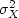.Given an initial estimate of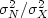,we see that equation (24) gives us X and (19) gives N, so that we can computeand.Presumably these computed values are better than our initial guesses. In statistics, the variances in equation (24) are called priors," and it makes sense to check them, and even more sense to correct them. From the corrected values we should be able to iterate, further improving the corrections. Equation (24) applies for each of the many frequencies, and there is only a single unknown, the ratio.Hence it seems as if we have plenty of information, and the bootstrapping procedure might work. A pessimist might call this bootstrapping a self-fulfilling prophecy, but we will see. What do you think?

Truth is stranger than fiction. I tried bootstrapping the variances. With my first starting value for the ratio,iterating led to the ratio being infinite. Another starting value led to the ratio being zero. All starting values led to zero or infinity. Eventually I deduced that there must be a metastable starting value. Perhaps the metastable value is the appropriate one, but I lack a rationale to assert it. It seems we cannot bootstrap the variances because the solutions produced do not tend to the correct variance, nor is the variance ratio correct. Philosophically, we can be thankful that these results failed to converge, since this outcome prevents us from placing a false confidence in the bootstrapping procedure.

 The variance of the solution to a least-squares problem is not usable to bootstrap to a better solution.

I conclude that linear-estimation theory, while it appears to be a universal guide to practice, is actually incomplete. Its incompleteness grows even more significant in later chapters, when we apply least squares to multivariate problems where the scalarbecomes a matrix. We continue our search for universal truth'' by studying more examples.

## EXERCISES:

1. Using the chain rule for differentiation, verify that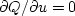and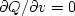is equivalent to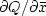, where x=u+iv.
2. Write code to verify the instability in estimating the variance ratio. 1 1Next: NONSTATIONARITY Up: HOW TO DIVIDE NOISY Previous: Explicit model for noise
Stanford Exploration Project
10/21/1998